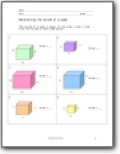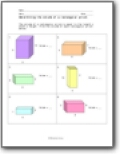# Volume Worksheets

#### How to Determine the Volume of a Cube and Rectangular Prism

A 3D shape has six indistinguishable square faces. A kick the bucket is a case of a 3D square. A rectangular crystal likewise has six appearances, yet its countenances can be squares as well as square shapes. A matchbox is a case of a rectangular crystal. Once in a while, it is simpler to see all the essences of a rectangular crystal or 3D square if we see its net. A net of a crystal is the figure acquired when cutting the crystal along a portion of its edges, unfurling it, and laying it level. In some cases, it is simpler to see all the essences of a rectangular crystal or 3D shape if we see its net. A net of a crystal is the figure gotten when cutting the crystal along a portion of its edges, unfurling it, and laying it level. Take a piece of paper and fold it over a matchbox, so it covers the entire box without going over a similar spot twice. Cut off additional paper pieces is essential, so you have just the paper that covers each facet of the matchbox. Straighten the paper and draw lines where the paper has been collapsed. Your sheet may appear as though one of the accompanying nets.###### Volume of a Cube

The volume of a cube is equal to the side x side x side. Find the volume of each cube below.###### Volume of a Rectangular Prism

The volume of a rectangular prism is equal to the length x width x height. Find the volume of each rectangular prism below.